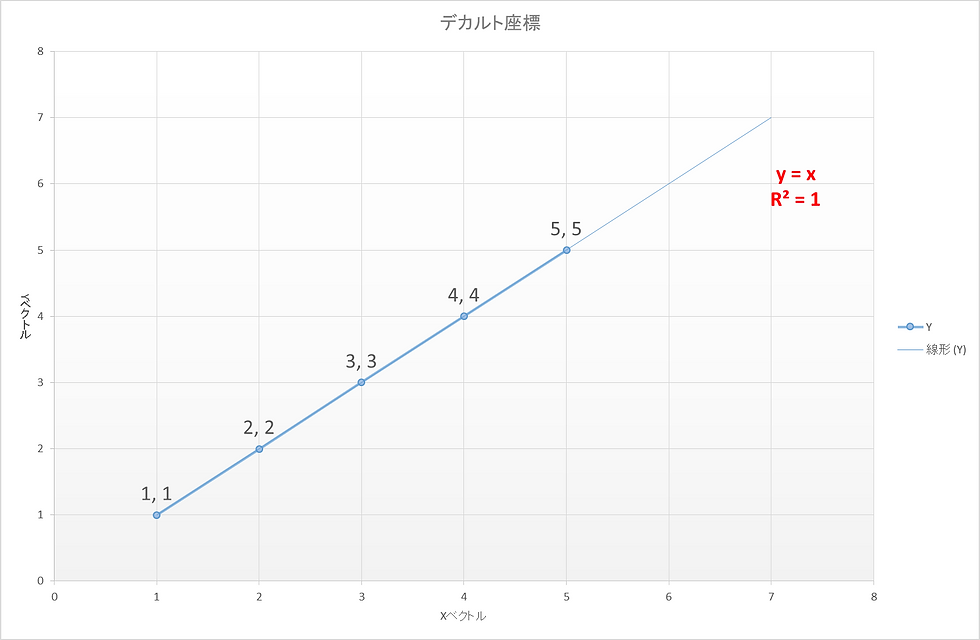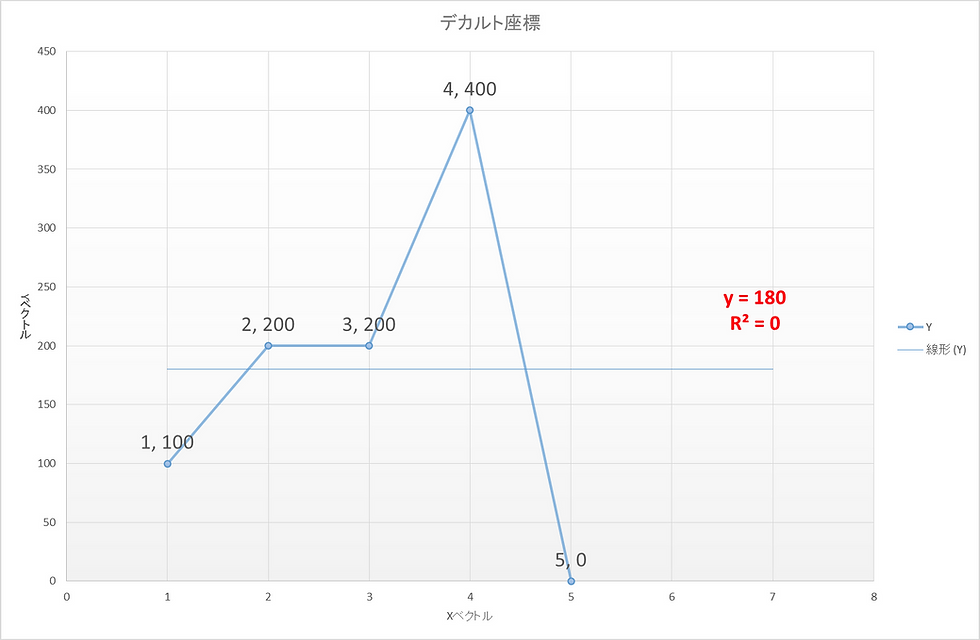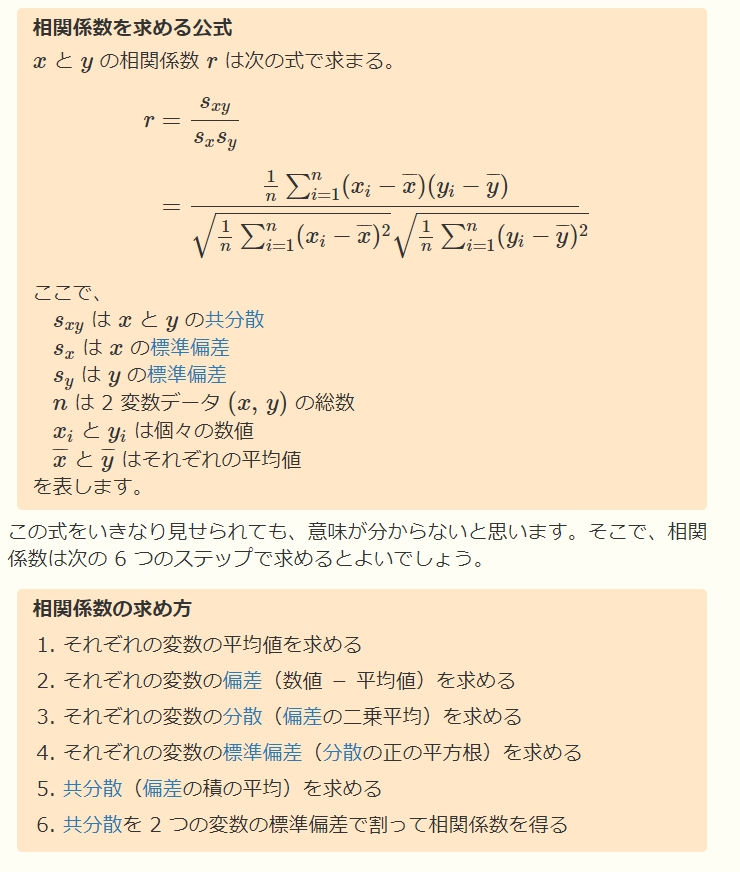top of page

• bookloveru2

# I tried to visualize and understand the correlation coefficient.

Hello everyone.

Today I'm going to describe [correlation coefficients].

Correlation coefficients are indicators of the relationship between two types of data.

The correlation coefficient takes a value from -1 to 1. The higher the number, the higher the relationship between the two types of data, i.e., the closer it is to 1, the higher the relationship between the two types of data.

Let's take a quick look at a simple example! (^^)!

First, we have two types of data.

In this case, we have two types of data, X and Y.

X contains 5 numbers (1,2,3,4,5).

Y also contains five numbers (1,2,3,4,5).

That is, X and Y have exactly the same value.

In this case, intuitively, without calculating, the data is the same, so the relationship between the two types of data is exactly the same, isn't it? And I feel.

That gut feeling is totally correct!

This means that the correlation coefficient is 1.

So, let's take a look at it.As shown in the above figure, we can see that the correlation coefficient is 1.Even in the linear model, you can see a straight line, where for every 1 increase in X, Y increases by 1.

The R^2 coefficient of determination is also 1, and everything (the relationship between the two types of data) can be explained by [Y=X]. (^_-)-☆.

Now let's look at the next case where the values of X and Y are completely opposite.

That is, two types of data with a correlation coefficient of -1.

X contains five numeric data, (1,2,3,4,5).

Y contains five numbers (5,4,3,2,1).

That is, X and Y are completely opposite values.As shown in the above figure, the correlation coefficient is -1.Even in the linear model, we can see a straight line, where for every 1 increase in X, Y increases by -1.

The R^2 coefficient of determination is also 1, which explains everything (the relationship between the two types of data) by [Y=-X+6]. (^_-)-☆

Finally, there is a case where X and Y are completely different numbers.

X contains five numbers (1,2,3,4,5).

Y contains five numbers (100,200,200,400,0).

That means X and Y are completely different numbers.As shown in the above figure, the correlation coefficient is zero.Even in the linear model, an increase in X does not necessarily mean an increase in Y. A straight line can be seen in the linear model.

The R^2 coefficient of determination is also zero, and a straight line appears that everything is [Y=180], which does not explain the relationship between the two types of data at all. ( ix)

Now, let's take a few applications.

Let's take a look at the correlation coefficients of stock prices in python.

Here's a look at 2018-12-1 to the present

We will compare the stock prices of Microsoft and Tesla.

To begin, we get the raw data from Yahoo Finance in the US.

As usual, I use the Google Collaboration to do this.

Apple and AMD are shown in the @param, but

Change it to Microsoft "MSFT" and Tesla "TSLA".

Then, here's the code.

Yes. Don!

```import datetime
import fix_yahoo_finance as yf
import matplotlib.pyplot as plt
import requests
import argparse
import numpy as np
import seaborn as sns
import pandas as pd

#スタート日を決める
number = "2018-12-1" #@param {type:"string"}

#銘柄コード
start = number
end = datetime.date.today()
code1 = "MSFT" #@param ["AAPL"] {allow-input: true}
code2 = "TSLA" #@param ["AMD"] {allow-input: true}

codelist = [code1,code2]
#終値取得(data2に終値を取り込み))
data2```

Execution Results.

This is for the period from 2018-12-1 to 2020-10-02.

Microsoft and Tesla stock prices, of which

Open Open, High, Low, Close, Adj Close and Volume.Next, the linear regression model and stock price trend graphs, basic statistics and correlation coefficient graphs are displayed.

```from sklearn import linear_model #線形モデル関係
import statsmodels.api as smf #統計量計算
X = data3["MSFT"]
Y = data3["TSLA"]
print(data3)
#単回帰分析モデルの作成
model = smf.OLS(X ,Y)
result = model.fit()
print(result.summary())
#描画
fig = plt.figure()
ax.plot(X)
ax.plot(Y, marker='^', linestyle='-.')
sns.pairplot(data3)

#データを対前日比の株価変動率に変換しstdpctに格納
stdpct = data3.pct_change().dropna() * 100

#株価変動率の基本統計量を取得
stastics = round(stdpct.describe(),2)

#統計量をグラフ化
fig, ax = plt.subplots(figsize=(8,6))
ax.axis('off')
ax.axis('tight')
ax.table(cellText=stastics.values,
colLabels=stastics.columns,
rowLabels=stastics.index,
loc='center',
bbox=[0,0,1,1])
#バイオリンプロット
plt.figure(figsize=(15, 6))
ax = sns.violinplot(data=stdpct, fliersize = 6, width = 1.6, inner=None, color="0.7", linewidth=0.3)
ax = sns.swarmplot(data=stdpct)
plt.grid(True)

#ここで、重ね過ぎたfigファイルがダブって、グラフが二重になるのを防ぐため、clf()で削除。
plt.clf()

#ヒートマップにて相関関係を表示
stdpct1 = stdpct.dropna()
sns.set(style="white")
#三角形の上半分をマスクする

Here, a beautiful violin plot is sacrificed, but

Never mind, I'll just explain the results.................First, from the raw data we just acquired, the stock's Adj Close "Adjusted Close

and stored in data3.

The result of a simple regression analysis is shown in the figure above.

The figure below shows a graph of the stock price trend over time.

The blue stock price is Microsoft.

The orange stock price is Tesla Inc.

Tesla Inc.'s 2020 implosion is well represented.

That 👇 is a scatter plot.And finally, the basic statistics and correlation coefficients.

Yes. Finally, the correlation coefficients are here.Here's an explanation of the basic statistic, but the values are converted to the rate of change.

count = number of stock prices (i.e. 461 days of stock price data)

Mean = Average

std=standard deviation

min=minimum value

25% = one quarter of a point in the quartile

50% = 2/4 points in the quartile

75% = 3/4 of a quartile

max=maximum value

It is.

Finally, finally, the correlation coefficient, which is the main part of this article.

The result was 0.48.

This means that the stock prices of Microsoft and Tesla have been

It turns out that there was a reasonably strong (slight) correlation ( ...) Memo Memo

summary

Correlation coefficient is a correlation coefficient that takes a value from -1 to 1, and the higher the number, i.e., the closer the number is to 1, the higher the relationship between the two types of data. It can be used as an indicator (*'o')

For more details on the calculation method, please refer to the other site 👇

The following diagram is from sci-pursuit.com.

sci-pursuit.com aims to provide science pursuits for those who want to pursue science at the elementary, middle and high school levels.

You can find it at: https://sci-pursuit.com/math/statistics/correlation-coefficient.htmlAnd that was it... ugh.

Okay, Bai-cha.

すべて表示
bottom of page